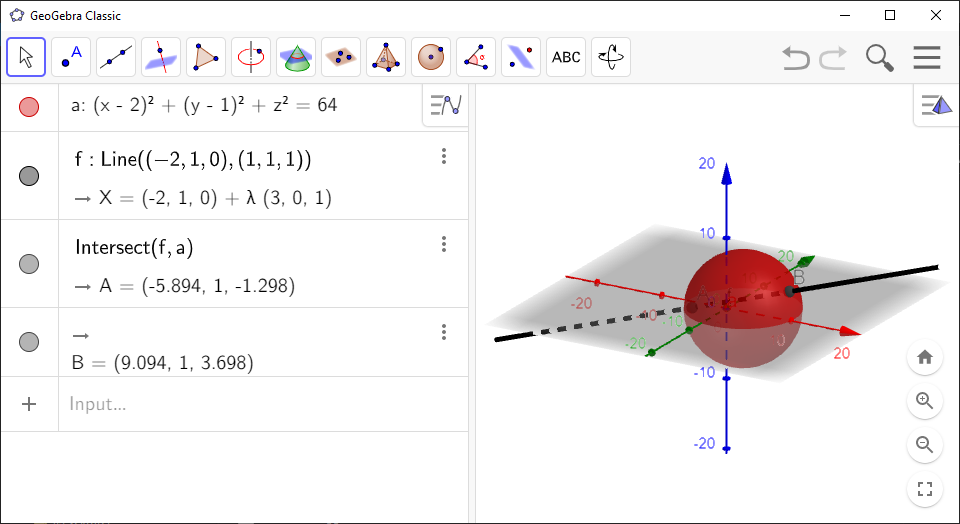# How to Find Intersection in GeoGebra

You can quickly find intersections between lines, planes and spheres in `GeoGebra`.

`GeoGebra` Instruction 1

1.
Open `3D Graphics` and `Algebra View` under`View` in`Menu`.
2.
Add the two objects that you want to intersect by entering them into `Algebra View`. If one or both of your objects are lines, then they must be added by using one of the `Line` commands.
3.
Enter

Intersect(<Object>, <Object>)

and fill in the names of the objects as they appear in `Algebra View`.

4.
The intersection points or lines are now shown in `Graphics View` and `Algebra View`.

Example 1

Enter the equation of the sphere

 ${\left(x-2\right)}^{2}+{\left(y-1\right)}^{2}+{z}^{2}=64$

in `Algebra View`. Create a line with the command `Line(<Point>, <Direction Vector>)`. Choose $\left(-2,1,0\right)$ as the point on the line, and $\phantom{\rule{-0.17em}{0ex}}\left(1,1,1\right)$ as the direction vector of the line. Then we use the command

Intersect(<Object>, <Object>)

to find where the line intersects the sphere.